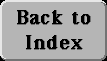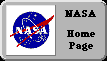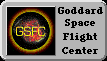# (21) Kepler's Third Law

Besides the Moon, Earth now has many artificial satellites, put up by us earthlings for a variety of purposes. The calculation applied by Newton to the Moon can also be used for them.

## Orbital velocity

Suppose the Earth were a perfect sphere of radius 1 RE = 6 317 000 meters and had no atmosphere. In principle, a satellite could then orbit just above its surface. The Earth would pull it downwards with a force F = mg, and because of the direction of this force, any accelerations would be in the up-down direction, too.

If the satellite is in a stable circular orbit and its velocity is V, then F supplies just the right amount of pull to keep the motion going. Which means

mg = F = mV2/ RE

Dividing both sides by m shows that the mass of the satellite does not matter, and leaves

V2/ RE = g

Multiplication of both sides by RE: gives

V2 = (g) (RE) = (9.81) (6 371 000) = 62 499 510 (m/ sec2)

V = 7905. 66 m/sec = 7.90566 km/s = VoThis is the velocity required by the satellite to stay in its orbit ("1" in the drawing). Any slower and it loses altitude and hits the Earth ("2"), any faster and it rises to greater distance ("3"). For comparison, a jetliner flies at about 250 m/sec, a rifle bullet at about 600 m/sec.

We again need a notation for square root. Since the HTML language does not provide one, we use the notation SQRT found in some computer languages. The square root of 2, for instance, can be written

SQRT(2) = 1. 41412. . .

If the speed V of our satellite is only moderately greater than Vo curve "3" will be part of a Keplerian ellipse and will ultimately turn back towards Earth. If however V is greater than 1. 4142. . .times Vo the satellite has attained escape velocity and will never come back: this comes to about 11.2 km/sec.

## Kepler's Third Law for Earth Satellites

The velocity for a circular Earth orbit at any other distance r is similarly calculated, but one must take into account that the force of gravity is weaker at greater distances, by a factor (RE/r)2. We then get

V2/ r = g (RE/ r)2 = g RE2/ r2

Let T be the orbital period, in seconds. Then (as noted earlier), the distance 2 pr covered in one orbit equals VT

>
 VT = 2 p r V = 2 p r/ T V2 = 4 p2r2/ T2 V2/ r = 4 p2r/ T2
 and by the earlier equality 4 p2r/ T2 = g RE2/ r2 Get rid of fractions by multiplying both sides by r2T2 4 p2r3 = g RE2 T2 To better see what we have, divide both sides by g RE2, isolating T2: T2 = (4p2/ g RE2) r3 What's inside the brackets is just a number. The rest tells a simple message--T2 is proportional to r3, the orbital period squared is proportional to the distance cubes. This is Kepler's 3rd law, for the special case of circular orbits around Earth. If you are not yet tired of the calculation, you may click here for turning the above into a practical formula.

Deriving a practical formula from Kepler's 3rd law.

Next Regular Stop: Frames of Reference: The BasicsAuthor and curator: David P. Stern, u5dps@lepvax.gsfc.nasa.gov
Last updated 24 August 1998